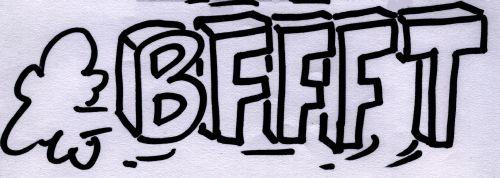# BFFFT (Blazingly Fast FFT)

## History

Discovered by the French mathematician Joseph Fourier in 1816, the forward and backward Fourier transforms (and its numerous variations) constitute, in a sense, the beginning of modern mathematics.

## FFT, a.k.a. Fast Fourier Transform

Today, Fourier transforms are performed by computers, on which the improved FFT (Fast Fourier Transform) algorithm has proven extremely valuable in many applications. The FFT of a given set of numbers can be defined recursively as the sum of two FFTs over odd and even points. Thus, unlike the direct implementation of Fourier's formulas, which are in O(n2), the FFT complexity is in O(nlog(n)).
The 2-dimensional FFT algorithm is also very simple. Using a simple array as input, a 2-dimensional Fast Fourier Transform consists of two one-dimensional FFTs performed (for instance) on each line first, and on each column next. Similar extentions exist in the case of dimension 3 or more.

## Computerizing the FFT

An important limitation, in FFT computer applications dealing with large arrays, is the fact that the algorithm is not well suited to parallelization, due to the huge amount of data that has to be transfered between computers. Since the transfers are proportional to the number of operations, network time soon becomes prominent when the number of processors is high.
A good alternative to parallelization does exists, however! FFT can be efficiently vectorized.

## BFFFT, a 2D Vectorized FFT

BFFFT is largely based on VFFT, a public domain code which is part of the CMLIB mathematical package. Paul Swarztrauber wrote 95% of it about 20 years ago. I only added a few subroutines on top of it, that can be useful if one needs to do 2-dimensional FFTs. VFFT only handles one-dimensional vectorized FFTs on arrays. BFFFT adds:
• 2-dimensional vectorized real-to-complex forward and backward subroutines,
• Fortran 90 language, while VFFT uses Fortran 77,
• double precision floats.
Note that BFFFT uses the OFFT utility. More information and sample instructions can be found in the beginning of the source file itself. Note that BFFFT is distributed under the GNU General Public License, you can use it, modify it and redistribute it under the conditions as mentioned in the license.
Contact: <romo661@free.fr>, my main page.